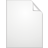CA+Calculator.xls Size : 16 Kb Type : xls

This has been tested on several characters all with noticable difference between their base damage and int.

A = base damage = ( strength + weapon damage ) x 1.AR / 2 [ for average

Ex. Str = 200, weapon damage = 300-310, AR = 10.

--------------------------------------------------------------------

A = (200+305) x 1.1

B = Int. CA = skill damage

-------------------------------------------------------------------------------------

Since getting this equation involves usage of log and answers were obtained by calculators, significant errors are also involved. There for two equations are listed: one over estimates, one underestimates, and the average should be very close ( usu with error of about 10 skill damage ).

-------------------------------------------------------------------------------------
Eq 1 : 0.05 x A x ( B ^ 0.779 ) x 1.SAR = CA1

Eq 2 : 0.065 x A x ( B ^ 0.732 ) x 1.SAR = CA2

( CA1 + CA2 ) / 2 is ur average CA. So ur skill damage should be within the range of CA-10 ------> CA +10.

----------------

How to get maximum skill damage : ( ONLY FOR MAX SKILL DAMAGE )

Also needs to be averaged and use basic calculus to find value of A and B.

Note that everyone has limited total points possible. Therefore

Str + Int = a constant for a normal character with fixed level.

Constant = C in this case.

-----------------------------------------------------------------------------------------

Eq1: 0.779 / ( (C+Average weapon damage ) x 1.AR ) ^ 0.221 - 1.779 x B ^ 0.779 = 0

Plug in your C and find your B, which is the points you should put your int as if you want max damage, then put rest points on str.

(C + Average weapon damage) x 0.779 / 1.779 = B1.

(C + Average weapon damage) x 0.732 / 1.732 = B2.

B1+B2 / 2 should be the int you want. then C-B = your str.

====================================================

How the first two equations were obtained.

By testing several characters. I found that : dA/A = dCA/CA

Since more str = more CA per point of Int, CA should be result of multiplication of Damage and Int.

Assuming 100 damage corresponds to 1 damage extra per pont of int.
Ex: 700 damage ( including weapon off course ) = 7 damage per point of int. ( this assumption is not applied in the equations )

therefore the equation takes the form of :

constant x A x B ^ n. n does not have to be whole number.

Then by comparing varies data, n is obtained and the constant as well.

Please leave feedback for wether it works.

Thank you very much.

Make a Free Website with Yola.